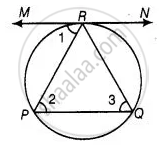# A chord PQ of a circle is parallel to the tangent drawn at a point R of the circle. Prove that R bisects the arc PRQ. - Mathematics

Sum

A chord PQ of a circle is parallel to the tangent drawn at a point R of the circle. Prove that R bisects the arc PRQ.

#### Solution

Given Chord PQ is parallel to tangent at R.

To prove: R bisects the arc PRQProof: ∠1 = ∠2  ....[Alternative interior angles]

∠1 = ∠3  ......[Angle between tangent and chord is equal to angle made by chord in alternative segment]

∴ ∠2 = ∠3

⇒ PR = QR  ......[Sides opposite to equal angles are equal]

⇒ PR = QR

So, R bisects PQ.

Concept: Concept of Circle - Centre, Radius, Diameter, Arc, Sector, Chord, Segment, Semicircle, Circumference, Interior and Exterior, Concentric Circles
Is there an error in this question or solution?

#### APPEARS IN

NCERT Mathematics Exemplar Class 10
Chapter 9 Circles
Exercise 9.3 | Q 8 | Page 107
Share